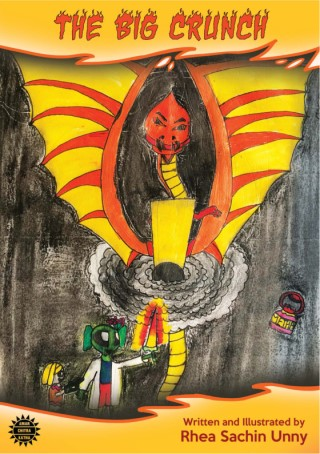## The Big Crunch(2)
• Description
• Author
• Info
• Reviews

### Description

 Jhaadoo is a young alien who wants to go away from his planet. But when the planet gets attacked by a vicious dragon, the fate of the Universe lies in the hands of this young alien and his friend. Will he be able to find the crucial blackhole and save the world. Read on to find out!

### Reviews

Mamatha B commented on The Big Crunch from The Big Crunch: A+++++++++++++++++++
Mamatha B commented on The Big Crunch from The Big Crunch: I.LOVE.DRAGONS!=2=2=2=2=2=2=2=2=2=2=2=2=2=2=2=2=2=2=2=2=2=2=2=2=2=2
Mamatha B loves The Big Crunch from The Big Crunch
Mamatha B likes The Big Crunch from The Big Crunch
Dhruti commented on The Big Crunch from The Big Crunch: F